# Kerala Syllabus 8th Standard Maths Solutions Chapter 7 Ratio

## Kerala Syllabus 8th Standard Maths Solutions Chapter 7 Ratio

Ratio Chapter Class 8 Kerala Syllabus Question 1.
In a regular polygon, the ratio of the inner and outer angle is 7 : 2. What is each angle? How many sides does the polygon
Solution:
Let the inner angle be 7x and the outer angle 2x .
Inner angle + outer angle = 180°
7x + 2x = 180°
9x = 180°
x = 180 ÷ 9 = 20°
Inner angle = 7x = 7 × 20 = 140°
Outer angle = 2x = 2 × 20 = 40°
Sum of the outer angles of a polygon of n sides is 360°. Since it is a regular polygon, the outer angles are equal,
n × 40 = 360°Number of sides of the polygon = 9

Hsslive Maths Class 8 Kerala Syllabus Question 2.
The number of girls and boys in a class are in the ratio 7 : 5 and there are 8 more girls than boys. How many girls and boys are there in this class?
Solution:
Let the number of girls be 7x and the number of boys be 5x ,
Difference = 7x – 5x = 2x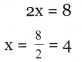Number of girls = 7x = 7 × 4 = 28
Number of boys = 5x = 5 × 4 = 20

Hsslive Class 8 Maths Kerala Syllabus Question 3.
Blue and yellow paints are mixed in the ratio 2 : 5 to make a new colour. 6 litres more of yellow 1 than blue is taken. How many litres of each is mixed?
Solution:
Let the measure of blue paint be 2x and yellow paint be 5x .
Their difference = 5x – 2x = 3x
3x = 6
x = 2
Quantity of blue paint = 2x = 2 × 2 = 4 litre
Quantity of yellow paint = 5x = 5 × 2 = 10 litre

Hsslive Guru 8th Class Maths Kerala Syllabus Question 4.
There are four right triangles, the ratio of perpendicular sides being 3 : 4 m each. One more fact about each is given below. Find the lengths of the sides of each triangle.
i. The difference in the lengths of the perpendicular sides is 24 metres.
ii. The hypotenuse is 24 metres.
iii. The perimeter is 24 metres.
iv. The area is 24 square metres.
Solution:
i. Let the perpendicular sides be 3x and 4x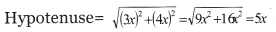The difference between the perpendicular sides be,
4x – 3x =x
x = 24
Sides are, 3x = 3 × 24 = 72 m
4x = 4 × 24 = 96 m
5x = 5 × 24 = 120 m

ii. Hypotenuse = 5x = 24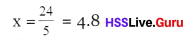Perpendicular sides 3x = 3 × 4.8 = 14.4 m
4x = 4 × 4.8 = 19.2 m

iii. Perimeter = 3x + 4x + 5x = 12x
12x = 24
x = 2
Sides are, 3x = 3 × 2 = 6m
4x = 4 × 2 = 8 m
5x = 5 × 2 = 10 m

iv. Area = 1/2 × 3x × 4x = 6x 2
6x 2 = 24
x 2 = 4; x = 2
Sides are, 3x = 3 × 2 = 6m
4x = 4 × 2 = 8m
5x = 5 × 2 = 10 m

Textbook Page No 137

8th Std Maths Guide Kerala Syllabus  Question 5.
Acid and water are mix ed in the ratio 4 : 3 to make a liquid. On adding 10 more litres of water, the ratio changed to 3 : 1. How many litres of acid and water does the liquid contain now?
Solution:
Let the volume of acid be 4x and volume of water be 3x volume of acid on adding 10 more litres of acid = 4x + 10 litres
present ratio = 3 : 1
4x + 10 : 3x = 3 : 1
3 × 3x = 1 × (4x + 10)
9x = 4x + 10
9x – 4x = 10 ; 5x = 10
x = 2
Volume of acid in the original mix ture = 4x = 4 × 2 = 8 litres
Volume of water in the original mix ture = 3x = 3 × 2 = 6 litres
Volume of acid in the present mix ture = 8 + 10 = 18 litres
Volume of water in the present mix ture = 6 litres

Hss Live Maths Class 8 Kerala Syllabus Question 6.
Two angles are in the ratio 1 : 2. On increasing the smaller angle by 6° and decreasing the larger angle by 6°, the ratio changed to 2 : 3. What were the original angle?
Solution:
Let x be the smaller angle and 2x be the larger angle,
x + 6 : 2x – 6 = 2 : 3
2(2x – 6) = 3 (x +6)
4x – 12 = 3x + 18
4x – 3x = 18 + 12 = 30; x = 30
Original smaller angle = x ° = 30°
Original larger angle =2x ° = 6o°

Hss Live Guru 8th Maths Kerala Syllabus Question 7.
The sides of a rectangle are in the ratio 4 : 5.
i. By what fraction should the shorter side be increased to make it a square?
ii. By what fraction should the longer side be decreased to make it a square?
Solution:
Let 4x be the smaller side and 5x be the larger side,
i. Let y part of a shorter side be added breadth after adding = 4x + 4xy = 4x (1 + y)
Ratio of breadth and length of a square = 1 : 1
so present ratio
4 × (1 + y) : 5x = 1 : 1
1 × 4x (1 + y) = 5x × 1
4(1 + y) = 5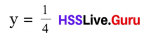On adding $$\frac{1}{4}$$ part of the original breadth, it can be made into square,

ii. Let y part of the longer side be substracted length after subtracting = 5x – 5xy
= 5x (1 – y)
As the present ratio is 1 : 1
5x (1 – y) : 4x = 1 : 1
1 × 5x (1 – y) = 1 × 4x
5 (1 – y) = 4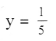On subtracting $$\frac{1}{5}$$ part of the original length, it can be made into square.

Hss Live Guru 8 Maths Kerala Syllabus Question 8.
Two quantities are in the ratio 3 : 5
i. If the smaller alone is made four times the original, What would be the ratio?
ii. If the smaller is doubled and the larger is halved, What would be the ratio?
Solution:
i. Let 3x be the smaller side and 5x be the larger side,
4 times the smaller = 4 × 3x = 12x
present ratio = 12x : 5x = 12 : 5

ii. Double the smaller = 2 × 3x = 6x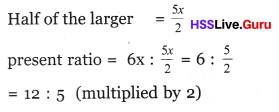Kerala Syllabus 8th Standard Maths Notes Question 9.
i. The capacities of two bottles are in the ratio 3 : 4. The smaller bottle was filled twice and the larger bottle was filled and emptied into a vessel. Twice the smaller and half the larger was emptied into another. What is the ratio of the quantities of water in the two vessels?

ii. In the problem, what is the capacities of the bottles are in the ratio 4 : 7?
Solution:
i. Let the capacity of the smaller bottle = 3x
the capacity of the larger bottle = 4x
Volume of water when the smaller bottle is filled twice = 2 × 3x = 6x
Volume of water when the larger bottle is filled once = 4x
Total volume now = 6x + 4x = 10x
Volume of the water when the smaller bottle is filled twice = 6x
Volume of water when larger bottle is filled half = $$\frac{4 x }{2}$$ = 2x
Total volume now = 6x + 2x = 8x
Ratio of the quantities of water = 10x : 8x , = 10 : 8 = 5 : 4

ii. Ratio of capacities of the bottle = 4 : 7
Let the capacity of the smaller bottle = 4x
the capacity of the larger bottle = 7x
Volume of water when the smaller bottle is filled twice = 2 × 4x = 8x
Volume of water when the larger bottle is filled once = 7x
Total volume now = 8x + 7x = 15x
Volume of the water when the smaller bottle is filled twice = 8x
Volume of water when larger bottle is filled half = $$\frac{7 x }{2}$$= 30 : 23 (multiplied by 2)

8th Std Maths Notes Kerala Syllabus Question 10.
The breadth and length of two rectangles are in the ratio 2 : 3. In another rectangle, whose breadth is 1 cm less and length is 3 cm less than those of the first, this ratio is 3 : 4. Calculate the breadth and length of both rectangles.
Solution:
Ratio between the breadth and length of the first rectangle = 2 : 3
Breadth of the first rectangle = 2x
Length of the first rectangle = 3x
Breadth of the second rectangle = 2x – 1
Length of the second rectangle = 3x – 3
Ratio between the breadth and length = 3 : 4
so, (2x – 1) : (3x – 3) = 3 : 4
3 (3x – 3) = 4 (2x – 1)
9x – 9 = 8x – 4
9x – 8x = -4 + 9
x = 5
Breadth of the first rectangle = 2x = 2 × 5 = 10cm
Length of the first rectangle = 3x = 3 × 5 = 15 cm
Breadth of the second rectangle = 2x – 1 = 10 – 1 = 9 cm
Length of the second rectangle = 3x – 3 = 15 – 3 = 12 cm

Textbook Page No 141

Hsslive Guru Class 8 Maths Kerala Syllabus Question 11.
Johny invested 50000 rupees, Jaleel 40000 rupees and Jayan 20000 rupees to start a business together. They got 3300 rupees as profit in a month, which they divided in the ratio of their investments. How much did each get ?
Solution:
Ratio of the investment = 50000 : 40000: 20000 = 5 : 4 : 2
Profit of one month = 3300 rupeesKerala Syllabus 8th Standard Notes Maths Question 12.
The capacities of three water tanks are in the ratio 2 : 3 : 5. The smallest of them can hold 2500 litres. How many litres can the other two hold ?
Solution:
Ratio of the capacities = 2 : 3 : 5
Let the capacities be 2x , 3x , 5x
Quantity of water in smaller tank = 2x = 2500
x = 1250 litres
Capacity of second tank = 3x = 3 × 1250 = 3750 litres
Capacity of third tank = 5x = 5 × 1250 = 6250 litres

Maths Guide For Class 8 Kerala Syllabus Question 13.
The angles of a triangle are in the ratio 1 : 3 : 5. How much is each angle?
Solution: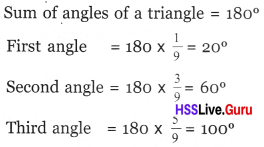Class 8 Maths Notes Kerala Syllabus Question 14.
The outer angles of triangle are in the ratio 5 : 6: 7. What are the angles ?
Solution:
Sum of outer angle = 360°
Let the angles be 5x , 6x , 7x
5x + 6x + 7x = 360°
18x = 360°
x = 20°
Angles are = 5x =5 x 20 = 100°
6x = 6 × 20 = 1200
7x = 7 × 20 = 140°

8th Standard Maths Notes Kerala SyllabusQuestion 15.
The sides of a triangle are in the ratio 2 : 3 : 4. The longest side is 20 cm more than the shortest side. Calculate the length of all three sides.
Solution:
Let sides be 2x , 3x , 4x then,
Since the longest side is 20 cm more than the shortest side,
4x = 2x + 20, 4x – 2x = 20
2x = 20
x = 10
First side = 2 × 10 = 20 cm
Second side = 3 × 10 = 30 cm
Third side = 4 × 10 = 40 cm

Class 8 Chapter 7 Maths Kerala Syllabus Question 16.
A box contains beads of three colors. Black beads and white beads are in the ratio 3 : 5. White and red beads are in the ratio 2 : 3. What is the ratio of all three colors ?
Solution: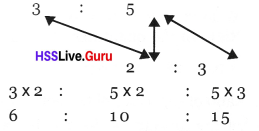Hsslive Guru 8 Maths Kerala Syllabus Question 17.
The length, breadth and height of a rectangle block are in the ratio 3 : 2 : 5 and its volume is 3750 cubic centimetres. Calculate the length, breadth and height.
Solution:
Let breadth be 3x , length be 2x and height be 5x , then
3x × 2x × 5x = 3750
30 × 3 = 37
x = 5
Breadth = 3x = 3 × 5 = 15 cm
Length = 2x = 2 × 5 = 10 cm
Height = 5x = 5 × 5 = 25 cm

Hss Live Guru Maths 8th Kerala Syllabus Question 1.
Sides of a triangular plot are in the ratio 3 : 5 : 7. Perimeter of the plot is 150 meter. Find the length of the sides.
Solution:
Perimeter = 150 m= 30 m, 50 m, 70 m

Question 2.
The denominator and numerator of fraction are in the ratio 3 : 1. If $$\frac{1}{2}$$ part of the numerator is added to it, prove that the ratio becomes 2 : 1.
Solution:Ratio between denominator and numerator, when $$\frac{1}{2}$$ part of the numerator is added to it, then the ratio is 6 : 3 = 2 : 1

Question 3.
Arun, Varun and Hari bought two packet of sweets, 245 in all. The ratio of the number of sweets Arun took to the number of sweets Varun took is 3 : 4. The ratio of the number of sweets Varun and Hari took is 6 : 7
How many sweets did each take?
Solution: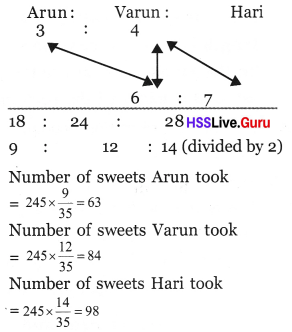Question 4.
In a goat farm, the ratio of the number of goats which give milk to the number of goats which don’t is 6 : 2. The number of goat which don’t give milk is 160. How many goats do give milk? And how many goats are there in all?
Solution:
Ratio of the number of goat which give milk to the number of goat which don’t = 6 : 2
We have to find how many times the number of goat which don’t give milk is the number of goats which give milk is $$\frac{6}{2}$$ times
that the number of goats which don’t give milk.
Therefore the number of goats which give milk $$160 \times \frac{6}{2}=480$$
Total number of goats = 160 + 480 = 640

Question 5.
Length and breadth of a rectangle are in the ratio 5 : 3. How many parts of the length of this rectangle is to be deducted so as to get the ratio of the length and breadth of the new rectangle is 7 : 6?
Solution:
5 : 3 = 10 : 6
If $$\frac{3}{10}$$ part of 10 is deducted from it, the ratio is 7 : 6
That is $$\frac{3}{10}$$ part of the length is deducted from it, the ratio is 7 : 6

Question 6.
In a school, the ratio of the number of boys to the number of teachers 2 : 3; and the ratio of the number of teacher to the number of girls is 4 : 5. What is the ratio of the number boys to girls.
Solution:
boys : teacher : girls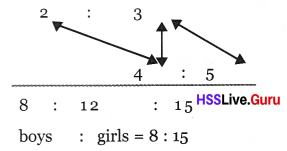Question 7.
The ratio between the number of boys and girls in a college is 8 : 5. If the number of girls is 800, find the total number of students.
Solution:
Let the number of boys = 8x
Let the number of girls = 5x
5x = 800
x = 800 ÷ 5 = 160
Number of boys = 8 × 160 = 1280
Total number of students = 800 + 1280 = 2080

Question 8.
The sides of a triangle are in the ratio 3 : 5 : 7. Its smallest side is 28 cm less than the largest side. Find the sides of the triangle.
Solution:
Ratio of the sides of the triangle = 3 : 5 : 7
3x = 7x – 28
7x – 28 = 3x
4x = 28
x = 28 ÷ 4
Smallest side = 3 × 7 = 21 cm
Second side = 5 × 7 = 35 cm
Largest side = 7 × 7 = 49 cm

Question 9.
To make a colour, red, blue and green paints mixed in the ratio 1 : 2 : 4. How much quantity red and green are needed for 10 bottle of blue paint?
Solution:
Let red paint be 1x bottle, blue paint
2x bottle and green paint 4x
2x = 10 x = 10 ÷ 2 = 5
Red paint needed = 1 × 5 = 5 bottles
Green paint needed = 4 × 5 = 20 bottles.

Question 10.
In a 50 litre mixture of milk and water, milk and water are in the ratio 3 : 2. How much more milk should be added to it to make the ratio as 3 : 1.
Solution:
Let the measure of milk = 3x litre and
that of water = 2x litre.
3x + 2x = 50, 5x = 50
x = 50 ÷ 5 = 10
Milk = 3 × 10 = 30 litres
Water = 2 × 10 = 20 litres
Let k more litre of milk is added to make the ratio 3 : 1
Then 30 + k : 20 = 3 : 1
30 + k = 60, k = 60 – 30 = 30
Measure of milk to be added = 30 litres

Question 11.
The length and breadth of a rectangle are in the ratio 8 : 5. The length is 9 centimetres more than the breadth. What are the length and breadth of the rectangle?
Solution:
Let the length of the rectangle = 8x and breadth = 5x
Their difference = 8x – 5x = 3x
This is given as 9 m, 8x – 5x = 9
3x = 9, x = 9 ÷ 3 = 3
∴ Length of the rectangle = 8 × 3 = 24 cm
Breadth of the rectangle = 5 × 3 = 15 cm

Question 12.
In a concrete, cement and sand are mixed in the ratio 4 : 3. Prove that double the quantity of cement should be added to it to make the ratio 4 : 1.
Solution:
cement : sand = 4 : 3
Let cement = 4x and sand = 3x .
Double of cement = 2 x 4x = 8x ,
Quantity of cement now = 4x + 8x = 12x
Quantity of sand = 3x
Their ratio now = 12x : 3x
= 12 : 3 (divided by x)
= 4 : 1 (divided by 3)
That is if double the quantity of cement is added, the ratio will become 4 : 1.

Question 13.
Ratio of the number of red beads and blackheads in a box is 6 : 5. If the number of red beads is 4 more than the number of black beads find the number of red beads and black beads in the box.
Solution:
Let the number of red beads = 6x
Number of black beads = 5x
6x – 5x = 1x ; = 6x – 5x = 4
This is given as 4. ∴ x = 4
Number of red beads = 6 × 4 = 24
Number of black beads = 5 × 4 = 20

Question 14.
The ratio of the number of boys to the number of girls in a school is 14 : 15. There are 27 more girls than boys. How many girls are there in this school? How many boys?
Solution:
Ratio of the number of boys to the number of girls = 14 : 15
Let girls be 15x & boys be 14x
Their difference = 15x – 14x
= x = 27
Number of girls = 15x = 15 × 27 = 405
Number of boys = 14x = 14 × 27 = 378

Question 15.
State that if the sides of a triangle are in the ratio 3 : 4 : 5, then it is a right angled triangle. If perimetre is 36 cm, then find the length of the sides?
Solution:
Sides are 3x , 4x and 5xSquare of two sides are equal to the square of the third side. Therefore, it is a right angled triangle.Question 16.
Ratio of cement, sand and gravel in a concrete mixture are in the ratio 1 : 3 : 4. If 80 kilogram of mixture is to be prepare, what quantity of cement, sand and gravel are to be taken?
Solution:
Let quantity of cement = x,
Quantity of sand = 2x,
Quantity of gravel = 4x
x + 3x + 4x = 80
8x = 8o ⇒ x = 10
quantity of cement = x = 10 kg
quantity of sand = 3x = 3 × 10 = 30 kg
quantity of gravel = 4x = 4 × 10 = 40 kg

Question 17.
Did the sides of a triangle be in the ratio 3 : 5 : 8 ? Why ?
Solution:
Sides are 3x, 5x and 8x .
3x + 5x = 8x
here sum of two sides is equal to the third side.
But sum of two sides should be greater than the third side. So the given sides
3x, 5x and 8x are not the sides of a triangle.
Therefore the ratio 3 : 5 : 8 cannot be the sides of a triangle.

Question 18.
In ∆ ABC, AB : BC = 2 : 3, BC : CA = 4 : 5, then find AB : BC : CA.
Solution: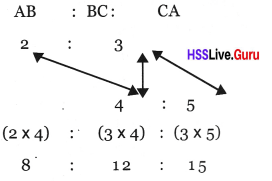Question 19.
One side of triangle given below is 12 cm. P is the midpoint of BC. Find the ratio of areas between ∆ ABC and ∆ APC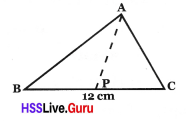Solution: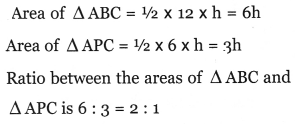Question 20.
Area of a rectangle is 2400 sq.cm. If length and breadth are in the ratio 8 : 3, find length and breadth? If length and breadth are increased by 20 cm, then find the ratio between length and breadth
Solution:
Length = 8x